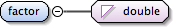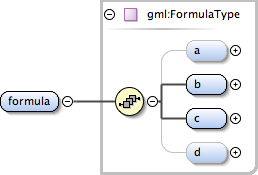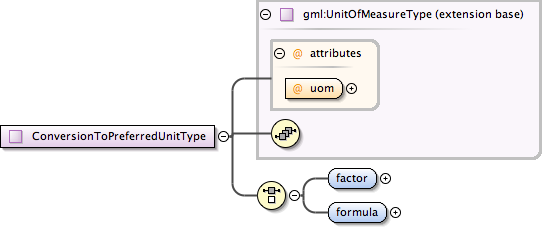### Showing:

 Annotations Attributes Diagrams Instances Properties Source Used by
Element gml:ConversionToPreferredUnitType / gml:factor
Namespace http://www.opengis.net/gml
Annotations
 `Specification of the scale factor by which a value using this unit of measure can be multiplied to obtain the corresponding value using the preferred unit of measure.`
DiagramType double
Properties
 content: simple
Source
 ``` Specification of the scale factor by which a value using this unit of measure can be multiplied to obtain the corresponding value using the preferred unit of measure. ```
Schema location http://schemas.opengis.net/gml/3.1.1/base/units.xsd
Element gml:ConversionToPreferredUnitType / gml:formula
Namespace http://www.opengis.net/gml
Annotations
 `Specification of the formula by which a value using this unit of measure can be converted to obtain the corresponding value using the preferred unit of measure.`
DiagramType gml:FormulaType
Properties
 content: complex
Model gml:a{0,1} , gml:b , gml:c , gml:d{0,1}
Children gml:a, gml:b, gml:c, gml:d
Instance
 ``` {0,1} {1,1} {1,1} {0,1} ```
Source
 ``` Specification of the formula by which a value using this unit of measure can be converted to obtain the corresponding value using the preferred unit of measure. ```
Schema location http://schemas.opengis.net/gml/3.1.1/base/units.xsd
Complex Type gml:ConversionToPreferredUnitType
Namespace http://www.opengis.net/gml
Annotations
 `Relation of a unit to the preferred unit for this quantity type, specified by an arithmetic conversion (scaling and/or offset). A preferred unit is either a base unit or a derived unit selected for all units of one quantity type. The mandatory attribute "uom" shall reference the preferred unit that this conversion applies to. The conversion is specified by one of two alternative elements: "factor" or "formula".`
DiagramType extension of gml:UnitOfMeasureType
Type hierarchy
Used by
 Elements gml:conversionToPreferredUnit, gml:roughConversionToPreferredUnit
Model gml:factor | gml:formula
Children gml:factor, gml:formula
Attributes
QName Type Fixed Default Use Annotation
uom anyURI required
 `Reference to a unit of measure definition, usually within the same XML document but possibly outside the XML document which contains this reference. For a reference within the same XML document, the "#" symbol should be used, followed by a text abbreviation of the unit name. However, the "#" symbol may be optional, and still may be interpreted as a reference.`
Source
 ``` Relation of a unit to the preferred unit for this quantity type, specified by an arithmetic conversion (scaling and/or offset). A preferred unit is either a base unit or a derived unit selected for all units of one quantity type. The mandatory attribute "uom" shall reference the preferred unit that this conversion applies to. The conversion is specified by one of two alternative elements: "factor" or "formula". Specification of the scale factor by which a value using this unit of measure can be multiplied to obtain the corresponding value using the preferred unit of measure. Specification of the formula by which a value using this unit of measure can be converted to obtain the corresponding value using the preferred unit of measure. ```
Schema location http://schemas.opengis.net/gml/3.1.1/base/units.xsd